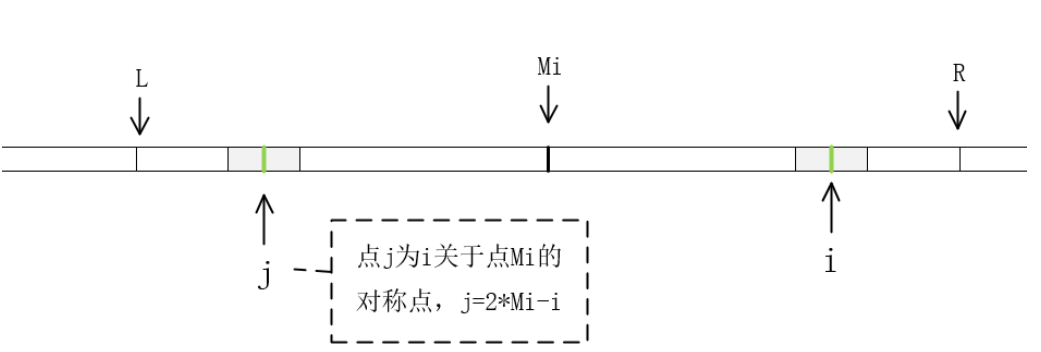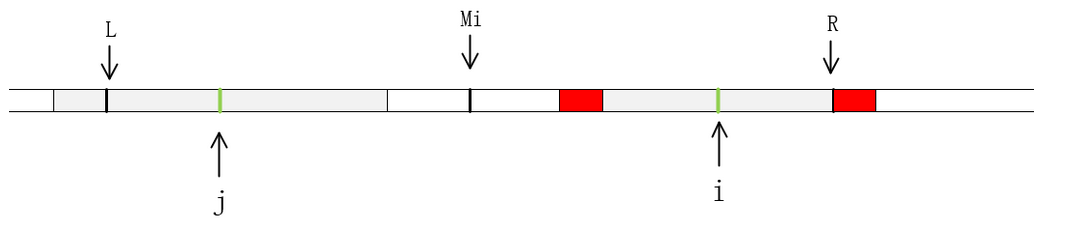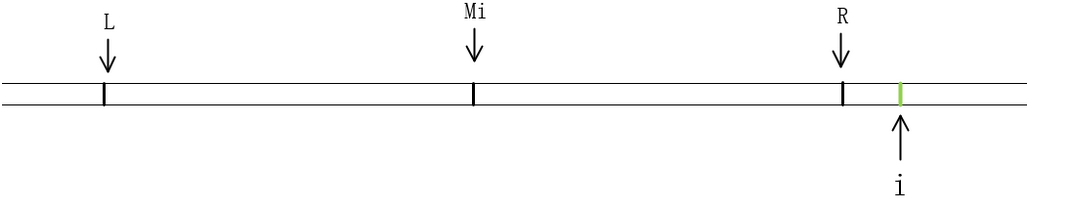# 回文数算法之马拉车算法

## April 4, 2020 • 算法

update time：2020,2,21

NOJP2132

## 什么是马拉车算法？

1975年，Manacher发明了Manacher Algorithm算法，俗称马拉车算法，这种算法能利用回文数的特性避免重复计算，该算法的时间复杂度仅仅只有O(n)

## 1.处理字符串

“abcd”，这是一个偶数长度的字符串，我们再字符串的字符间隙中先插入“#”，然后再在开头和结尾分别插入“@”，“&”，然后我们便可以得到一个新的字符串“@#a#b#a#b#&”，他的长度为11，是一个奇数长度的字符串

“abc",这是一个奇数长度的字符串，我们按照相同的方法处理他，就得到了新的字符串"@#a#b#c#&”，长度为9，也是一个奇数长度的字符串

## 2.计算最长回文子串数组P

### 2.什么是最长回文子串数组P

index0123456789101112
T@#a#b#a#b#a#@
P0010305030100

### 3.如何计算数组P

i和j相对称，所以`j=2*Mi-i`a. 当i<=R，并且P[j]<R-i 时，说明以点j为中心的回文串并没有超出范围[L,R]，则由回文串的特性可以得知：P[i]=P[j]b.当i<=R，并且P[j]>=R-i，即如上图所示，当我们还以为P[i]=P[j]的时候，问题出现了，如果P[i]=P[j]，那么是不是P[L-1]=P[R+1]呢？这很显然是不对的，因为R和L分别是以Mi为中心的回文字符串的最大回文边界，如果P[L-1]=P[R+1]，那么L和R便不再是最大回文边界了，出现了矛盾点，所以P[L-1]必不可能等于P[R+1]，对于这种情况，我们还是只能从R+1开始一 一匹配，知道失配为止，从而更新R和对应的Mi以及P[i]c.当i>R时，此时我们无法再利用回文串的特性，只能老老实实的一步步取匹配

## 3.总结一下这个算法

1. 处理字符串
2. 分情况制作P数组
3. 遍历P数组，计算结果

``````S="ababa"
#初始字符串
A = '@#' + '#'.join(S) + '#\$'
Z =  * len(A)
print(list(A))
#处理字符串并输出
center = right = 0
for i in range(1, len(A) - 1):
if i < right:
Z[i] = min(right - i, Z[2 * center - i])
#2 * center - i对应的是j的位置
while A[i + Z[i] + 1] == A[i - Z[i] - 1]:
Z[i] += 1
#对应第二种情况
if i + Z[i] > right:
center, right = i, i + Z[i]
#移动对称中心
print(Z)
print(sum((v+1)//2 for v in Z))
``````

``````#include<bits/stdc++.h>
using namespace std;
int Z;
int main(){
string str1;
while(cin>>str1){
memset(Z,0, sizeof(Z));
string A="@#";
for (int i=0;i<str1.length();i++){
A+=str1[i];
A+="#";
}
A+="\$";
int center=0,right=0;
for (int i=1;i<A.length()-1;i++){
if (i<right)
Z[i]=min(right-i,Z[2*center-i]);
while (A[i+Z[i]+1]==A[i - Z[i] - 1]){
Z[i]++;
}
if (i + Z[i] > right) {
center = i;
right = i + Z[i];
}
}
int ans=0;
for (int x=0;x<A.length();x++){
ans+=(Z[x]+1)/2;
}
cout<<ans<<endl;
}

return 0;
}
``````